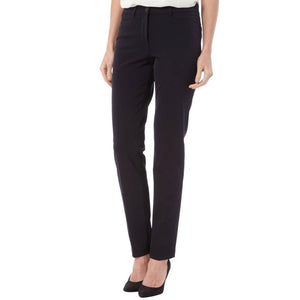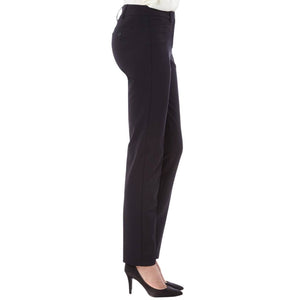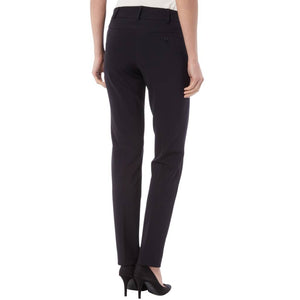cambio

# Renira Long

Regular price \$295 Unit price  per

Shipping calculated at checkout.
• 88% Polyamide 12% elasthan
• Machine wash cold
• Hang to dry
• Mid rise
• Excellent fit
• Fits long
• Slim pant

Size Guide

• 34 = 4 = 25
• 36 = 6 = 26
• 38 = 8 = 28
• 40 = 10 = 30
• 42 = 12 = 31
• 44 = 14 = 42
• 46 = 16 = 33